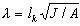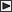■建築研究報告

 圧縮と曲げを受ける木材の強度に関する研究 久田　俊彦 建築研究報告　No.12,　昭和29年2月

 ＜概要＞ There have been many studies on wood columns under centric axial load but few reports on wood members subjected to combined compressive and bending stresses. 　The present design formula for wood members under combined stresses has been adopted in every country as a conventional from of combining compressive stress with bending one, therefore the adaptability and safety of the formulas of this type are not sufficiently clarified. 　In this investigation, the author has made some experiments on wood columns subjected to centric and eccentric loads and also to combined centric and lateral loads. The test results have been compared with the theoretical values introduced by assuming stress-strain relationship of the material. After comparisons, it has been recognized that the results showed good agreement with values given by the proposed theory. Furthermore, according to those results, the propriety of Study on Wood Members Subjected to Combined Compressive and Bending Stresses the conventional formula recommended in Japanese Standard for Calculation of Wood Structure has been discussed and criticized of its safety. 　The notations used in this paper are as follows: b = width of a rectangular column h = depth of a rectangular column, measured in the direction of eccentricity or of side load A = b×h = area of the cross section of a rectangular column e = eccentricity e' = distance from geometrical center axis to elastic center axis α0 = initial deflection at the midlength of a column δ= deflection at the midlength of a column lk = buckling length of a column or unsupported length, i.e., distance from upper knife edge to lower one= slenderness ratio of a column J = moment of inertia of the cross section of a column Z = section modulus of the cross section of a column P = axial compressive load Pmax = maximum value of axial compressive load H = lateral load acting at the midlength of a column Report of the Building Research Institute M = bending moment induced by direct acting side load or eccentric end moment σc = Pmax/A = maximum direct compressive stress induced by Pmax σb = M/Z = bending stress induced in the outer fiber of a column by M κ=σb/σc Ft = unit failing stress in tension of the column material Fc = unit failing stress in compression of the column material (parallel to grain) E = Young's modulus of elasticity of the column material ne = experimental value of σc/ Fc nr = theoretical value of σc/ Fc fc = allowable unit compressive stress fb = allowable unit bending stress w = specific gravity of the column material for air dried condition ψ= water content of the column material所在地・交通案内関連リンクサイトマップお問い合わせリンク・著作権